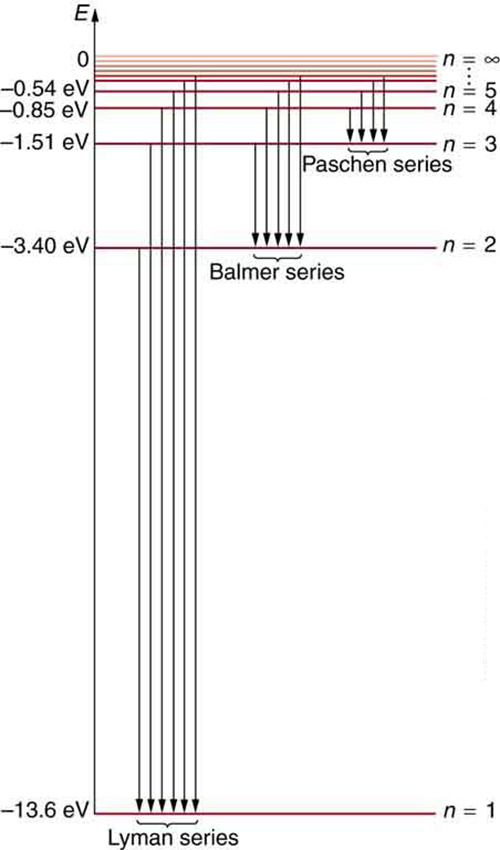# 30.3 Bohr’s theory of the hydrogen atom  (Page 5/14)

 Page 5 / 14
${E}_{n}=\frac{1}{2}{m}_{e}{v}^{2}-k\frac{{\text{Zq}}_{e}^{2}}{{r}_{n}}.$

Now we substitute ${r}_{n}$ and $v$ from earlier equations into the above expression for energy. Algebraic manipulation yields

${E}_{n}=-\frac{{Z}^{2}}{{n}^{2}}{E}_{0}\left(n=\text{1, 2, 3, ...}\right)$

for the orbital energies of hydrogen-like atoms    . Here, ${E}_{0}$ is the ground-state energy $\left(n=1\right)$ for hydrogen $\left(Z=1\right)$ and is given by

${E}_{0}=\frac{{2\pi }^{2}{q}_{e}^{4}{m}_{e}{k}^{2}}{{h}^{2}}=\text{13.6 eV.}$

Thus, for hydrogen,

${E}_{n}=-\frac{\text{13.6 eV}}{{n}^{2}}\text{(}n=\text{1, 2, 3, ...).}$

[link] shows an energy-level diagram for hydrogen that also illustrates how the various spectral series for hydrogen are related to transitions between energy levels.Energy-level diagram for hydrogen showing the Lyman, Balmer, and Paschen series of transitions. The orbital energies are calculated using the above equation, first derived by Bohr.

Electron total energies are negative, since the electron is bound to the nucleus, analogous to being in a hole without enough kinetic energy to escape. As $n$ approaches infinity, the total energy becomes zero. This corresponds to a free electron with no kinetic energy, since ${r}_{n}$ gets very large for large $n$ , and the electric potential energy thus becomes zero. Thus, 13.6 eV is needed to ionize hydrogen (to go from –13.6 eV to 0, or unbound), an experimentally verified number. Given more energy, the electron becomes unbound with some kinetic energy. For example, giving 15.0 eV to an electron in the ground state of hydrogen strips it from the atom and leaves it with 1.4 eV of kinetic energy.

Finally, let us consider the energy of a photon emitted in a downward transition, given by the equation to be

$\Delta E=\text{hf}={E}_{\text{i}}-{E}_{\text{f}}.$

Substituting ${E}_{n}=\left(–\text{13.6 eV}/{n}^{2}\right)$ , we see that

$\text{hf}=\left(\text{13.6 eV}\right)\left(\frac{1}{{n}_{\text{f}}^{2}}-\frac{1}{{n}_{\text{i}}^{2}}\right).$

Dividing both sides of this equation by $\text{hc}$ gives an expression for $1/\lambda$ :

$\frac{\text{hf}}{\text{hc}}=\frac{f}{c}=\frac{1}{\lambda }=\frac{\left(\text{13.6 eV}\right)}{\text{hc}}\left(\frac{1}{{n}_{\text{f}}^{2}}-\frac{1}{{n}_{\text{i}}^{2}}\right).$

It can be shown that

$\left(\frac{\text{13.6 eV}}{\text{hc}}\right)=\frac{\left(\text{13.6}\phantom{\rule{0.25em}{0ex}}\text{eV}\right)\left(1.602×{\text{10}}^{\text{−19}}\phantom{\rule{0.25em}{0ex}}\text{J/eV}\right)}{\left(6.626×{\text{10}}^{\text{−34}}\phantom{\rule{0.25em}{0ex}}\text{J·s}\right)\left(2.998×{\text{10}}^{8}\phantom{\rule{0.25em}{0ex}}\text{m/s}\right)}=1.097×{\text{10}}^{7}\phantom{\rule{0.25em}{0ex}}{\text{m}}^{–1}=R$

is the Rydberg constant    . Thus, we have used Bohr’s assumptions to derive the formula first proposed by Balmer years earlier as a recipe to fit experimental data.

$\frac{1}{\lambda }=R\left(\frac{1}{{n}_{\text{f}}^{2}}-\frac{1}{{n}_{\text{i}}^{2}}\right)$

We see that Bohr’s theory of the hydrogen atom answers the question as to why this previously known formula describes the hydrogen spectrum. It is because the energy levels are proportional to $1/{n}^{2}$ , where $n$ is a non-negative integer. A downward transition releases energy, and so ${n}_{i}$ must be greater than ${n}_{\text{f}}$ . The various series are those where the transitions end on a certain level. For the Lyman series, ${n}_{\text{f}}=1$ — that is, all the transitions end in the ground state (see also [link] ). For the Balmer series, ${n}_{\text{f}}=2$ , or all the transitions end in the first excited state; and so on. What was once a recipe is now based in physics, and something new is emerging—angular momentum is quantized.

## Triumphs and limits of the bohr theory

Bohr did what no one had been able to do before. Not only did he explain the spectrum of hydrogen, he correctly calculated the size of the atom from basic physics. Some of his ideas are broadly applicable. Electron orbital energies are quantized in all atoms and molecules. Angular momentum is quantized. The electrons do not spiral into the nucleus, as expected classically (accelerated charges radiate, so that the electron orbits classically would decay quickly, and the electrons would sit on the nucleus—matter would collapse). These are major triumphs.

#### Questions & Answers

what is physics
Rhema Reply
a15kg powerexerted by the foresafter 3second
Firdos Reply
what is displacement
Xolani Reply
movement in a direction
Jason
hello
Hosea
Explain why magnetic damping might not be effective on an object made of several thin conducting layers separated by insulation? can someone please explain this i need it for my final exam
anas Reply
Hi
saeid
hi
Yimam
What is thê principle behind movement of thê taps control
Oluwakayode Reply
while
Hosea
what is atomic mass
thomas Reply
this is the mass of an atom of an element in ratio with the mass of carbon-atom
Chukwuka
show me how to get the accuracies of the values of the resistors for the two circuits i.e for series and parallel sides
Jesuovie Reply
Explain why it is difficult to have an ideal machine in real life situations.
Isaac Reply
tell me
Promise
what's the s . i unit for couple?
Promise
its s.i unit is Nm
Covenant
Force×perpendicular distance N×m=Nm
Oluwakayode
İt iş diffucult to have idêal machine because of FRİCTİON definitely reduce thê efficiency
Oluwakayode
if the classica theory of specific heat is valid,what would be the thermal energy of one kmol of copper at the debye temperature (for copper is 340k)
Zaharadeen Reply
can i get all formulas of physics
BPH Reply
yes
haider
what affects fluid
Doreen Reply
pressure
Oluwakayode
Dimension for force MLT-2
Promise Reply
what is the dimensions of Force?
Osueke Reply
how do you calculate the 5% uncertainty of 4cm?
melia Reply
4cm/100×5= 0.2cm
haider
how do you calculate the 5% absolute uncertainty of a 200g mass?
melia Reply
= 200g±(5%)10g
haider
use the 10g as the uncertainty?
melia
which topic u discussing about?
haider
topic of question?
haider
the relationship between the applied force and the deflection
melia
sorry wrong question i meant the 5% uncertainty of 4cm?
melia
its 0.2 cm or 2mm
haider
thank you
melia
Hello group...
Chioma
hi
haider
well hello there
sean
hi
Noks
hii
Chibueze
10g
Olokuntoye
0.2m
Olokuntoye
hi guys
thomas

### Read also:

#### Get Jobilize Job Search Mobile App in your pocket Now!

Source:  OpenStax, College physics. OpenStax CNX. Jul 27, 2015 Download for free at http://legacy.cnx.org/content/col11406/1.9
Google Play and the Google Play logo are trademarks of Google Inc.

Notification Switch

Would you like to follow the 'College physics' conversation and receive update notifications?ByByBy Robert MorrisBy OpenStaxBy Abby SharpByBy Anonymous UserBy Yacoub JayoghliBy OpenStaxBy OpenStaxBy DanielrosenbergerBy OpenStax